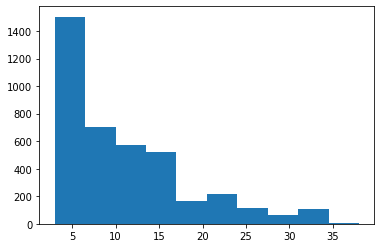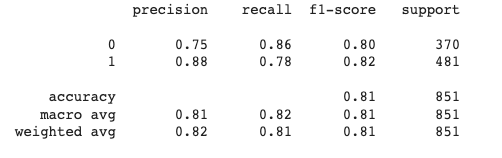GFG App
Open AppBrowser
Continue

# Fine-tuning BERT model for Sentiment Analysis

Google created a transformer-based machine learning approach for natural language processing pre-training called Bidirectional Encoder Representations from Transformers. It has a huge number of parameters, hence training it on a small dataset would lead to overfitting. This is why we use a pre-trained BERT model that has been trained on a huge dataset. Using the pre-trained model and try to “tune” it for the current dataset, i.e. transferring the learning, from that huge dataset to our dataset, so that we can “tune” BERT from that point onwards.

In this article, we will fine-tune the BERT by adding a few neural network layers on our own and freezing the actual layers of BERT architecture. The problem statement that we are taking here would be of classifying sentences into POSITIVE and NEGATIVE by using fine-tuned BERT model.

## Preparing the dataset

The sentence column has text and the label column has the sentiment of the text – 0 for negative and 1 for positive. We first load the dataset followed by, some preprocessing before tuning the model.

## Python

 `import` `pandas as pd ` `import` `numpy as np ` `df ``=` `pd.read_csv(``'/content/data.csv'``)`

### Split dataset:

After loading the data, split the data into train, validation ad test data. We are taking the 70:15:15 ratio for this division. The inbuilt function of sklearn is being used below to split the data. We use stratified attributes to ensure that the proportion of the categories remains the same after splitting the data.

## Python

 `from` `sklearn.model_selection ``import` `train_test_split ` ` `  `train_text, temp_text, train_labels, temp_labels ``=` `train_test_split(df[``'sentence'``], df[``'label'``],  ` `                                                                    ``random_state ``=` `2021``,  ` `                                                                    ``test_size ``=` `0.3``,  ` `                                                                    ``stratify ``=` `df[``'label'``]) ` ` `  ` `  `val_text, test_text, val_labels, test_labels ``=` `train_test_split(temp_text, temp_labels,  ` `                                                                ``random_state ``=` `2021``,  ` `                                                                ``test_size ``=` `0.5``,  ` `                                                                ``stratify ``=` `temp_labels)`

### Load pre-trained BERT model and tokenizer

Next, we proceed with loading the pre-trained BERT model and tokenizer. We would use the tokenizer to convert the text into a format(which has input ids, attention masks) that can be sent to the model.

## Python

 `#load model and tokenizer ` `bert ``=` `AutoModel.from_pretrained(``'bert-base-uncased'``) ` `tokenizer ``=` `BertTokenizerFast.from_pretrained(``'bert-base-uncased'``) `

If we take the padding length as the maximum length of text found in the training texts, it might leave the training data sparse. Taking the least length would in turn lead to loss of information. Hence, we would plot the graph and see the “average” length and set it as the padding length to trade-off between the two extremes.

## Python

 `train_lens ``=` `[``len``(i.split()) ``for` `i ``in` `train_text] ` `plt.hist(train_lens)`From the graph above, we take 17 as the padding length.

### Tokenizing the data

Tokenize the data and encode sequences using the BERT tokenizer.

## Python

 `# tokenize and encode sequences  ` `tokens_train ``=` `tokenizer.batch_encode_plus( ` `    ``train_text.tolist(), ` `    ``max_length ``=` `pad_len, ` `    ``pad_to_max_length ``=` `True``, ` `    ``truncation ``=` `True` `) ` ` `  `tokens_val ``=` `tokenizer.batch_encode_plus( ` `    ``val_text.tolist(), ` `    ``max_length ``=` `pad_len, ` `    ``pad_to_max_length ``=` `True``, ` `    ``truncation ``=` `True` `) ` ` `  `tokens_test ``=` `tokenizer.batch_encode_plus( ` `    ``test_text.tolist(), ` `    ``max_length ``=` `pad_len, ` `    ``pad_to_max_length ``=` `True``, ` `    ``truncation ``=` `True` `) ` ` `  `train_seq ``=` `torch.tensor(tokens_train[``'input_ids'``]) ` `train_mask ``=` `torch.tensor(tokens_train[``'attention_mask'``]) ` `train_y ``=` `torch.tensor(train_labels.tolist()) ` ` `  `val_seq ``=` `torch.tensor(tokens_val[``'input_ids'``]) ` `val_mask ``=` `torch.tensor(tokens_val[``'attention_mask'``]) ` `val_y ``=` `torch.tensor(val_labels.tolist()) ` ` `  `test_seq ``=` `torch.tensor(tokens_test[``'input_ids'``]) ` `test_mask ``=` `torch.tensor(tokens_test[``'attention_mask'``]) ` `test_y ``=` `torch.tensor(test_labels.tolist())`

## Defining the model

We first freeze the BERT pre-trained model, and then add layers as shown in the following code snippets:

## Python

 `#freeze the pretrained layers ` `for` `param ``in` `bert.parameters(): ` `    ``param.requires_grad ``=` `False` ` `  `#defining new layers ` `class` `BERT_architecture(nn.Module): ` ` `  `    ``def` `__init__(``self``, bert): ` `       `  `      ``super``(BERT_architecture, ``self``).__init__() ` ` `  `      ``self``.bert ``=` `bert  ` `       `  `      ``# dropout layer ` `      ``self``.dropout ``=` `nn.Dropout(``0.2``) ` `       `  `      ``# relu activation function ` `      ``self``.relu ``=`  `nn.ReLU() ` ` `  `      ``# dense layer 1 ` `      ``self``.fc1 ``=` `nn.Linear(``768``,``512``) ` `       `  `      ``# dense layer 2 (Output layer) ` `      ``self``.fc2 ``=` `nn.Linear(``512``,``2``) ` ` `  `      ``#softmax activation function ` `      ``self``.softmax ``=` `nn.LogSoftmax(dim``=``1``) ` ` `  `    ``#define the forward pass ` `    ``def` `forward(``self``, sent_id, mask): ` ` `  `      ``#pass the inputs to the model   ` `      ``_, cls_hs ``=` `self``.bert(sent_id, attention_mask``=``mask, return_dict``=``False``) ` `       `  `      ``x ``=` `self``.fc1(cls_hs) ` ` `  `      ``x ``=` `self``.relu(x) ` ` `  `      ``x ``=` `self``.dropout(x) ` ` `  `      ``# output layer ` `      ``x ``=` `self``.fc2(x) ` `       `  `      ``# apply softmax activation ` `      ``x ``=` `self``.softmax(x) ` ` `  `      ``return` `x`

Also, add an optimizer to enhance the performance:

## Python

 `optimizer ``=` `AdamW(model.parameters(),lr ``=` `1e``-``5``)  ``# learning rate`

Then compute class weights, and send them as parameters while defining loss function to ensure imbalance in the dataset is handled well while computing the loss.

## Training the model

After defining the model, define a function to train the model (fine-tune, in this case):

## Python

 `# function to train the model ` `def` `train(): ` `   `  `  ``model.train() ` ` `  `  ``total_loss, total_accuracy ``=` `0``, ``0` `   `  `  ``# empty list to save model predictions ` `  ``total_preds``=``[] ` `   `  `  ``# iterate over batches ` `  ``for` `step,batch ``in` `enumerate``(train_dataloader): ` `     `  `    ``# progress update after every 50 batches. ` `    ``if` `step ``%` `50` `=``=` `0` `and` `not` `step ``=``=` `0``: ` `      ``print``(``'  Batch {:>5,}  of  {:>5,}.'``.``format``(step, ``len``(train_dataloader))) ` ` `  `    ``# push the batch to gpu ` `    ``batch ``=` `[r.to(device) ``for` `r ``in` `batch] ` `  `  `    ``sent_id, mask, labels ``=` `batch ` ` `  `    ``# clear previously calculated gradients  ` `    ``model.zero_grad()         ` ` `  `    ``# get model predictions for the current batch ` `    ``preds ``=` `model(sent_id, mask) ` ` `  `    ``# compute the loss between actual and predicted values ` `    ``loss ``=` `cross_entropy(preds, labels) ` ` `  `    ``# add on to the total loss ` `    ``total_loss ``=` `total_loss ``+` `loss.item() ` ` `  `    ``# backward pass to calculate the gradients ` `    ``loss.backward() ` ` `  `    ``torch.nn.utils.clip_grad_norm_(model.parameters(), ``1.0``) ` ` `  `    ``# update parameters ` `    ``optimizer.step() ` ` `  `    ``# model predictions are stored on GPU. So, push it to CPU ` `    ``preds``=``preds.detach().cpu().numpy() ` ` `  `    ``# append the model predictions ` `    ``total_preds.append(preds) ` ` `  `  ``# compute the training loss of the epoch ` `  ``avg_loss ``=` `total_loss ``/` `len``(train_dataloader) ` `   `  `  ``# predictions are in the form of (no. of batches, size of batch, no. of classes). ` `  ``total_preds  ``=` `np.concatenate(total_preds, axis``=``0``) ` ` `  `  ``#returns the loss and predictions ` `  ``return` `avg_loss, total_preds`

Now, define another function that would evaluate the model on validation data.

## Python

 `# code ` `print` `"GFG"` `# function for evaluating the model ` `def` `evaluate(): ` `   `  `  ``print``(``"\nEvaluating..."``) ` `   `  `  ``# deactivate dropout layers ` `  ``model.``eval``() ` ` `  `  ``total_loss, total_accuracy ``=` `0``, ``0` `   `  `  ``# empty list to save the model predictions ` `  ``total_preds ``=` `[] ` ` `  `  ``# iterate over batches ` `  ``for` `step,batch ``in` `enumerate``(val_dataloader): ` `     `  `    ``# Progress update every 50 batches. ` `    ``if` `step ``%` `50` `=``=` `0` `and` `not` `step ``=``=` `0``: ` `       `  `      ``# # Calculate elapsed time in minutes. ` `      ``# elapsed = format_time(time.time() - t0) ` `             `  `      ``# Report progress. ` `      ``print``(``'  Batch {:>5,}  of  {:>5,}.'``.``format``(step, ``len``(val_dataloader))) ` ` `  `    ``# push the batch to gpu ` `    ``batch ``=` `[t.to(device) ``for` `t ``in` `batch] ` ` `  `    ``sent_id, mask, labels ``=` `batch ` ` `  `    ``# deactivate autograd ` `    ``with torch.no_grad(): ` `       `  `      ``# model predictions ` `      ``preds ``=` `model(sent_id, mask) ` ` `  `      ``# compute the validation loss between actual and predicted values ` `      ``loss ``=` `cross_entropy(preds,labels) ` ` `  `      ``total_loss ``=` `total_loss ``+` `loss.item() ` ` `  `      ``preds ``=` `preds.detach().cpu().numpy() ` ` `  `      ``total_preds.append(preds) ` ` `  `  ``# compute the validation loss of the epoch ` `  ``avg_loss ``=` `total_loss ``/` `len``(val_dataloader)  ` ` `  `  ``# reshape the predictions in form of (number of samples, no. of classes) ` `  ``total_preds  ``=` `np.concatenate(total_preds, axis``=``0``) ` ` `  `  ``return` `avg_loss, total_preds`

## Test the data

After fine-tuning the model, test it on the test dataset. Print a classification report to get a better picture of the model’s performance.

## Python

 `# get predictions for test data ` `with torch.no_grad(): ` `  ``preds ``=` `model(test_seq.to(device), test_mask.to(device)) ` `  ``preds ``=` `preds.detach().cpu().numpy() ` `   `  `from` `sklearn.metrics ``import` `classification_report ` `pred ``=` `np.argmax(preds, axis ``=` `1``) ` `print``(classification_report(test_y, pred))`

After testing, we would get the results as follows:Classification report